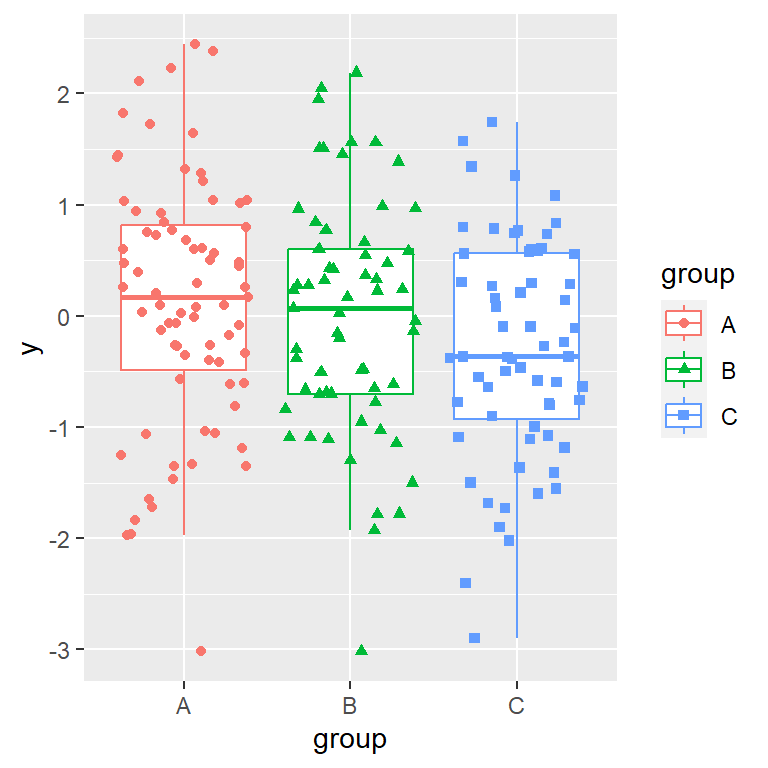# Box plot with jittered data points in ggplot2

## Box plot with jittered points with `geom_jitter`

Adding jittered points (a stripchart) to a box plot in ggplot is useful to see the underlying distribution of the data. You will need to use `geom_jitter`.

``````# install.packages("ggplot2")
library(ggplot2)

# Data
set.seed(8)
y <- rnorm(200)
df <- data.frame(y)

# Basic box plot
ggplot(df, aes(x = "", y = y)) +
geom_boxplot() +
geom_jitter()``````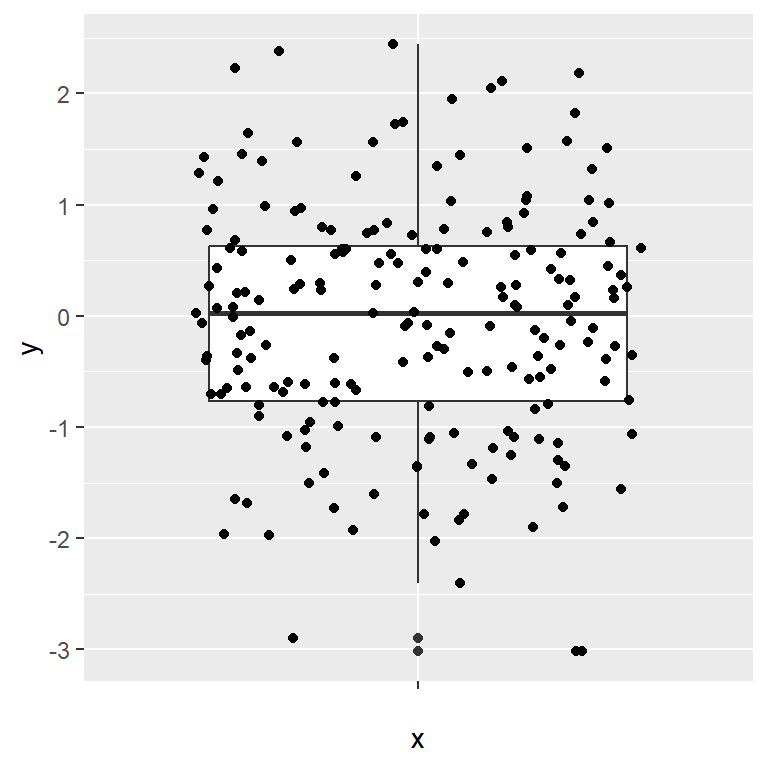A good practice is removing the outliers of the box plot with `outlier.shape = NA`, as the jitter will add them again.

``````# install.packages("ggplot2")
library(ggplot2)

# Data
set.seed(8)
y <- rnorm(200)
df <- data.frame(y)

# Basic box plot
ggplot(df, aes(x = "", y = y)) +
geom_boxplot(outlier.shape = NA) +
geom_jitter()``````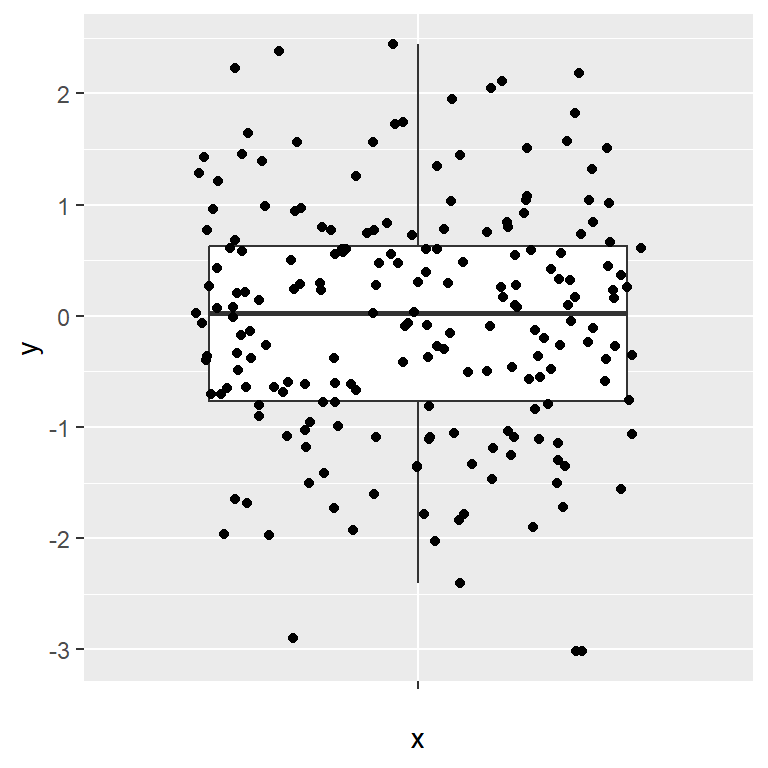Recall that you can flip the axes with `coord_flip` or flipping the variables inside `aes`.

``````# install.packages("ggplot2")
library(ggplot2)

# Data
set.seed(8)
y <- rnorm(200)
df <- data.frame(y)

# Basic box plot
ggplot(df, aes(x = "", y = y)) +
geom_boxplot(outlier.shape = NA) +
geom_jitter() +
coord_flip()``````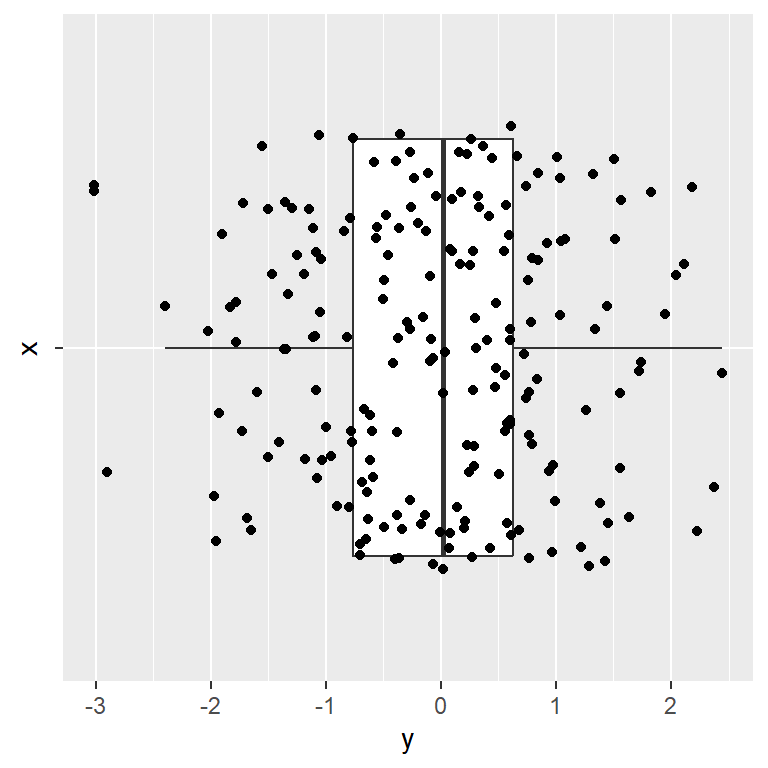## Customization of the jittered points

The jittered points can be customized in several ways. You can change the amount of jitter with `width`, the color of the points, its shape or its size, as shown in the examples below.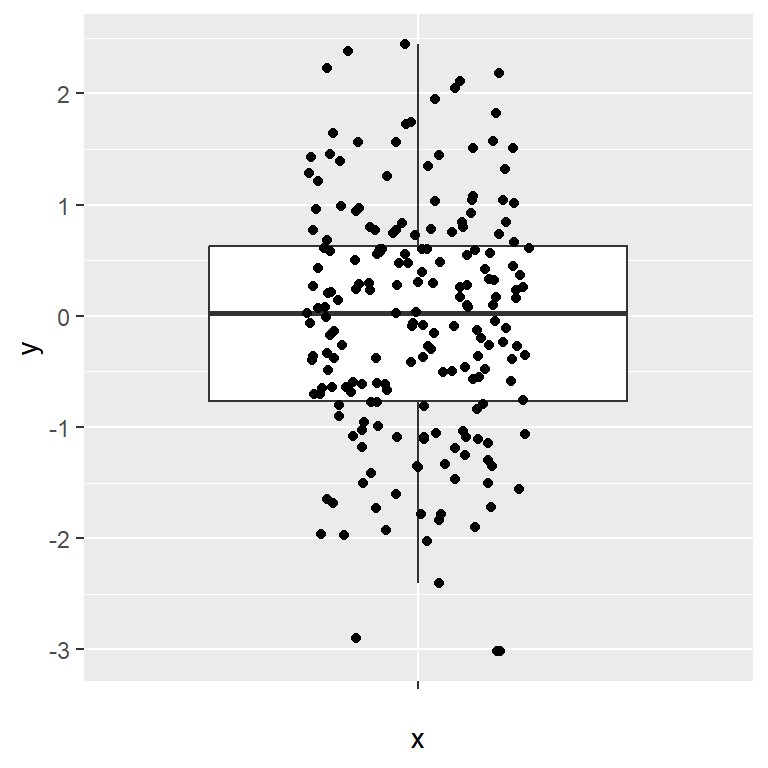Width

``````# install.packages("ggplot2")
library(ggplot2)

# Data
set.seed(8)
y <- rnorm(200)
df <- data.frame(y)

# Basic box plot
ggplot(df, aes(x = "", y = y)) +
geom_boxplot(outlier.shape = NA) +
geom_jitter(width = 0.2)``````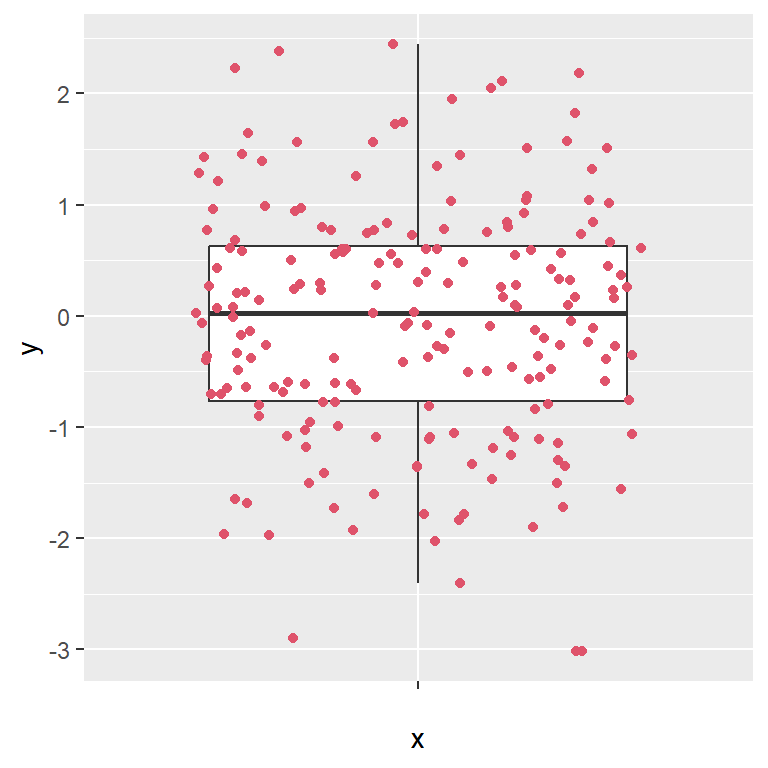Colour

``````# install.packages("ggplot2")
library(ggplot2)

# Data
set.seed(8)
y <- rnorm(200)
df <- data.frame(y)

# Basic box plot
ggplot(df, aes(x = "", y = y)) +
geom_boxplot(outlier.shape = NA) +
geom_jitter(colour = 2)``````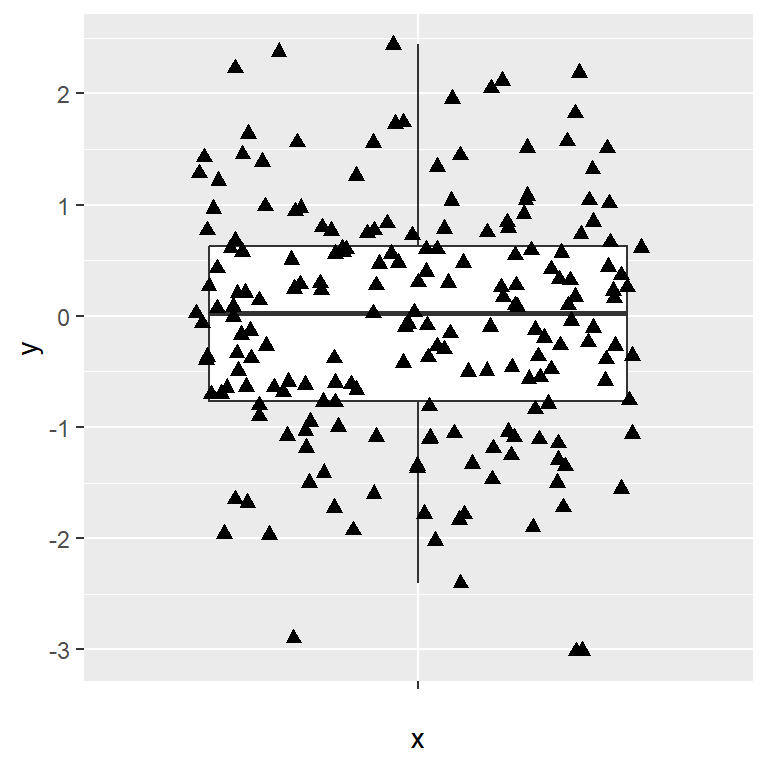Shape and size

``````# install.packages("ggplot2")
library(ggplot2)

# Data
set.seed(8)
y <- rnorm(200)
df <- data.frame(y)

# Basic box plot
ggplot(df, aes(x = "", y = y)) +
geom_boxplot(outlier.shape = NA) +
geom_jitter(shape = 17, size = 2)``````

## Box plot by group with jitter

If you have a categorical variable representing groups you can create a box plot by group and add the points to each group and customize its color, size and shape.

Jitter by group

``````# install.packages("ggplot2")
library(ggplot2)

# Data
set.seed(8)
y <- rnorm(200)
group <- sample(LETTERS[1:3], size = 200,
replace = TRUE)
df <- data.frame(y, group)

# Box plot by group with jitter
ggplot(df, aes(x = group, y = y)) +
geom_boxplot(outlier.shape = NA) +
geom_jitter()``````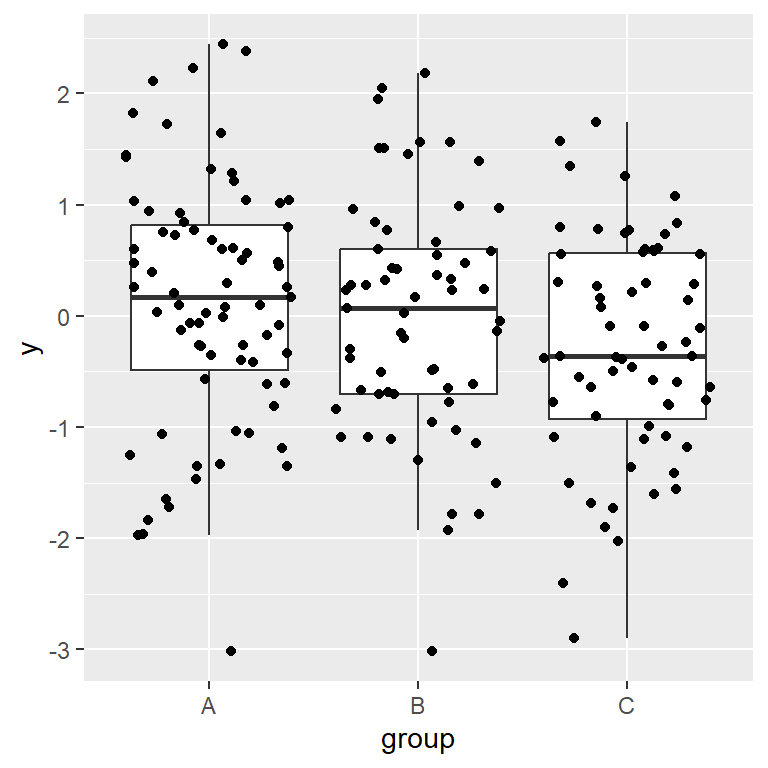Jitter by group with custom color

``````# install.packages("ggplot2")
library(ggplot2)

# Data
set.seed(8)
y <- rnorm(200)
group <- sample(LETTERS[1:3], size = 200,
replace = TRUE)
df <- data.frame(y, group)

# Box plot by group with jitter
ggplot(df, aes(x = group, y = y)) +
geom_boxplot(outlier.shape = NA) +
geom_jitter(colour = 2)``````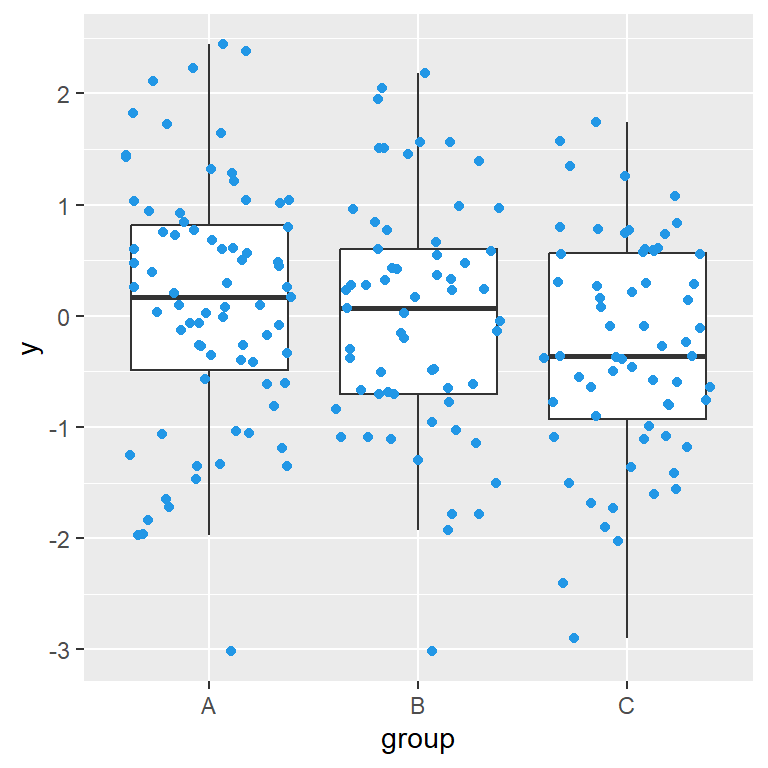Jitter by group with color by group

``````# install.packages("ggplot2")
library(ggplot2)

# Data
set.seed(8)
y <- rnorm(200)
group <- sample(LETTERS[1:3], size = 200,
replace = TRUE)
df <- data.frame(y, group)

# Box plot by group with jitter
ggplot(df, aes(x = group, y = y, colour = group)) +
geom_boxplot(outlier.shape = NA) +
geom_jitter()``````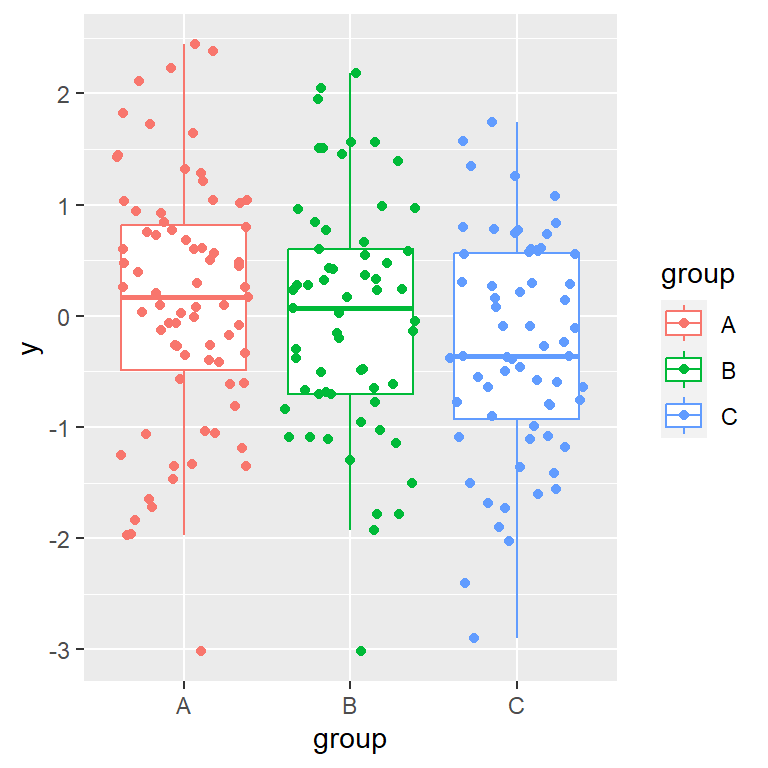Jitter by group with custom shape

``````# install.packages("ggplot2")
library(ggplot2)

# Data
set.seed(8)
y <- rnorm(200)
group <- sample(LETTERS[1:3],
size = 200,
replace = TRUE)
df <- data.frame(y, group)

# Box plot by group with jitter
ggplot(df, aes(x = group, y = y,
colour = group,
shape = group)) +
geom_boxplot(outlier.shape = NA) +
geom_jitter()``````